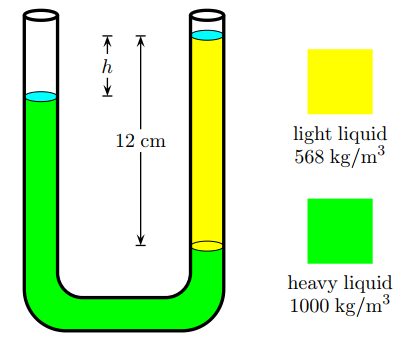# Problem: A simple U-tube that is open at both ends is partially filled with a heavy liquid of density 1000 kg/m3 . A liquid of density 568 kg/m3 is then poured into one arm of the tube, forming a column 12 cm in height, as shown. What is the difference in the heights of the two liquid surfaces? A. 5.54 B. 1.8582 C. 1.7507 D. 1.0318 E. 2.4066 F. 5.184 G. 9.288 H. 3.3512 I. 2.954 J. 5.97

90% (53 ratings)
###### Problem Details

A simple U-tube that is open at both ends is partially filled with a heavy liquid of density 1000 kg/m3 . A liquid of density 568 kg/m3 is then poured into one arm of the tube, forming a column 12 cm in height, as shown.

What is the difference in the heights of the two liquid surfaces?

A. 5.54

B. 1.8582

C. 1.7507

D. 1.0318

E. 2.4066

F. 5.184

G. 9.288

H. 3.3512

I. 2.954

J. 5.97Frequently Asked Questions

What scientific concept do you need to know in order to solve this problem?

Our tutors have indicated that to solve this problem you will need to apply the Pressure Gauge concept. If you need more Pressure Gauge practice, you can also practice Pressure Gauge practice problems.

How long does this problem take to solve?

Our expert Physics tutor, Juan took 3 minutes and 48 seconds to solve this problem. You can follow their steps in the video explanation above.

What professor is this problem relevant for?

Based on our data, we think this problem is relevant for Professor Gazes' class at CHICAGO.